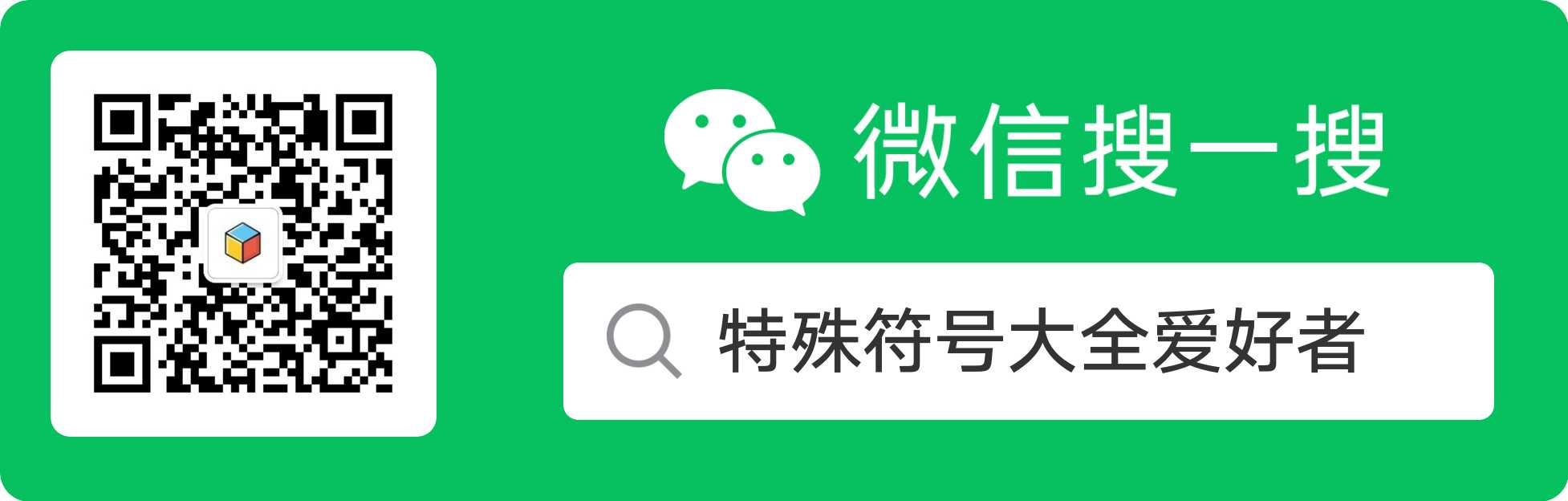# 火星文符号大全

⊙▂⊙ ⊙0⊙ ⊙＾⊙ ⊙ω⊙ ⊙﹏⊙ ⊙△⊙ ⊙▽⊙

`?▂? ?0? ?＾? ?ω? ?﹏? ?△?  ?▽? ∩▂∩ ∩0∩ ∩＾∩ ∩ω∩ ∩﹏∩ ∩△∩ ∩▽∩ ●▂●  ●0●  ●＾●  ●ω●  ●﹏●  ●△●  ●▽● ∪▂∪ ∪0∪ ∪＾∪ ∪ω∪ ∪﹏∪ ∪△∪ ∪▽∪ ≥▂≤ ≥0≤ ≥＾≤ ≥ω≤ ≥﹏≤ ≥△≤ ≥▽≤ ＞▂＜ ＞0＜ ＞＾＜ ＞ω＜ ＞﹏＜ ＞△＜ ＞▽＜ ╯▂╰ ╯0╰ ╯＾╰ ╯ω╰ ╯﹏╰ ╯△╰ ╯▽╰ ＋▂＋ ＋0＋ ＋＾＋ ＋ω＋ ＋﹏＋ ＋△＋ ＋▽＋ ˋ▂ˊ ˋ0ˊ ˋ＾ˊ ˋωˊ ˋ﹏ˊ ˋ△ˊ ˋ▽ˊ ˇ▂ˇ ˇ0ˇ ˇ＾ˇ ˇωˇ ˇ﹏ˇ  ˇ△ˇ ˇ▽ˇ ˙▂˙ ˙0˙ ˙＾˙ ˙ω˙ ˙﹏˙ ˙△˙ ˙▽˙ ≡(▔﹏▔)≡ ⊙﹏⊙‖∣° ˋ＾ˊ〉-# ╯＾╰〉 (=｀′=) <(｀^′)>  o(?""?)o (ˉ▽ˉ；) (-__-)b ＼ ＿ ／   ￣□￣｜｜  ------\(˙<>˙)/------   <("""O""")> (#｀′)凸  (｀▽′)ψ  （°ο°）~ @   (^人^) (O ^ ~ ^ O)  (＊?↓˙＊) [>/<] ↓。υ。↓  (*^〔^*)  ( /。 )   ( ' – ' ) ( ^3^ )╱~~  (；°○° ) ( > c < ) ＝＝＝＝＝＝＝＝＝＝＝＝＝＝＝＝＝＝＝＝＝＝＝＝＝＝＝       ||||| 动物篇 ||||| <。)#)))≤ 烤鱼 (??)nnn 毛毛虫 (0^◇^0)/ 麻雀 <*)>> >=< 鱼骨头 (=^ ^=) 猫 /(*w*) 兔子 ≡[。。]≡ 螃蟹 (ˉ(∞)ˉ) 猪 ■D" 咖啡杯 (:≡ 水母 (。。)～ 蝌蚪 ε==3 骨头 <□:≡ 乌贼 <｀▽′> 老虎 ○●○— 烤丸子 (:◎)≡ 章鱼 ζ。≡ 狮子 (●-●) 太阳眼镜 @/" 蜗牛 ∑^)/ 乌鸦 --<-<-<@ 玫瑰花 (((●< 蟑螂 (=^ω^=) 狐狸 ＜※ 花束 ＝＝＝＝＝＝＝＝＝＝＝＝＝＝＝＝＝＝＝＝＝＝＝＝＝＝＝ ||||| 杂物篇 ||||| ■D″咖啡杯 ∠※ 花束   8＜ 小剪刀 ＝＝＝＝＝＝＝＝＝＝＝＝＝＝＝＝＝＝＝＝＝＝＝＝＝＝＝   ||||| 满意.满足 <(￣）￣)> []~(￣▽￣)~* (￣﹏￣) (￣ˇ￣) (￣）￣)> <(￣）￣)/ (｀﹏′) ╮(｀▽′)╭ (｀▽′)/ ============================ ||||| 万岁.真棒.开心~ ≥▽≤y (/≥▽≤/) (≥▽≤)y (/≥▽≤)/~┴┴ (≥▽≤)(@) (≥3≤)/ (≥ω≤)/ o(≥ω≤)o o(≥o≤)o ˋ( ° ▽、° ) o(≥v≤)o ┴┴~(≥▽≤)/~┴┴ ≥□≤○ ============================ ||||| 惊讶.吃惊~ 《⊙＿⊙《 (⊙＿⊙) (@口@) ∑(@)(￣?￣)+ ∑(⊙▽⊙"a (@[]@!!) ㄟ(≥◇≤)ㄏ(￣ε(#￣) ∑( ° △ °|||)︴ =========================== ||||| 无奈.装傻~ (￣▽￣)~* (￣▽￣)" ╮(╯▽╰)╭ ╮(￣▽￣)╭ =￣ω￣= (￣3￣)a ￣▽￣ ╮(╯3╰)╭ ╮(╯3╰)╭ ╮(╯_╰)╭ (= 0 =)y o(）＾）)o (￣.￣) (￣< ￣)> (～o￣▽￣)～o ~。。。(~￣▽￣)~[] ˋ(′～｀)ˊ └(^o^)┘; 偶头好状壮ㄋㄟ``` ﹌○﹋ 喔嗨呦^ˇ^≡ =^_^= 温馨的微笑!!! Y(^_^)Y 举双手胜利 ^o^/ 欢呼 ^o^y 胜利^o^y ↖(^ω^)↗ 小猪为你打气！ ~^o^~ 加油呦! *^?^* 笑，打 *^ο^* 哦～，呵呵傻笑 *^◎^* 呵呵大笑（嘴唇好厚） *^÷^* 得意的笑（有上下唇的哟） ~~~^_^~~~ 笑毙啰（笑得连眼泪都蹦出来了...） (-.-)=3 松ㄌ一口气~ ~~~///(^v^)\~~~ 微笑表示友善!!!~哈~哈~ ~@^_^@~ 可爱呦! \*^o^*// 可爱ㄋㄟ~ ~*.*~ 害羞又迷人的小女生 #^_^# 脸红了!! ∩__∩y 耶~~^^ (装可爱?!) （*^＠^*） 乖～（还含个奶嘴哦） X﹏X糟糕．． 完蛋的意思呀~~ (° ο°)~@ 晕倒了.. {{{(>_<)}}} 发抖 ╯﹏╰ 粉无奈~~ (╯-╰)/ 很没劲/无耐的意思 （╯＾╰〉 一脸苦瓜 ｝_｝ 粉无奈..粉悲情 -____-" 唉~~别提了..... 。_。 受到打击，表情呈现呆滞样～ （*@＾@*〉 悲，晕 -（- 好伤心. //(ㄒoㄒ)// 流泪中... ::>_<:: 哭 T=T鸣~~我在哭... %>_<% 我要哭了哦... ╰_╯我发火了!! 生气的意思~~~眉毛都翘起来~~ >_<# 粉生气~冒青筋 @x@ 生气 （ˋ＾ˊ〉-# 生气 （＞﹏＜） 不～ （*+﹏+*）~ @ 受不了～ x__x, 唉..... 别哭了! >_<||| 很尴尬~!!! ^_^; 尴尬 ⊙﹏⊙‖∣° 真尴尬～～ ^_^||| 好尴尬！ ^_^" 尴尬的笑..... →_→ 怀疑的眼神~~ ..@_@|||||.. 头昏眼花 …(⊙_⊙;)… ○圭~○列~~怎么酱？ o_o .... 沉思(说穿了"呆滞状况") O__O" 呆滞的眼神~~~!! ///^_^....... 造成"冷"...干笑~ ?o?||| 听无(就是听不懂ㄉ意思啦^^) ( )? 什么意思?? (+_+)? 不知道你在什么 （?ε?）? 哩公啥哇听无啦~ o_O??? 发生啥事? @_@a 搔头，疑惑 一 一+ 锐利的眼神~ >"<|||| 伤脑筋的意思~ ｀(*>﹏<*)′ 好刺激.. （*@ο@*） 哇～ O_o 惊讶~~~ （⊙o⊙） 目瞪口呆 （°ο°）~ @ 晕倒，不省人事 *@_@* 崇拜的眼神;眼睛为之一亮 ★~★ 见到偶像眼睛为之一亮 ^◎- 爱你呦~~ (*^?^*) 啵一个! (^_^)∠※ 送你一束花 （*^〔^*〕 羞羞 ｀（*^﹏^*）′ 超级羞羞 ｀（*∩_∩*）′ 献上 ~_~ 想睡觉了的意思!! (～ o ～)~zZ 我想睡啦～ π_π? 打瞌睡 =@~@= 一个看到美眉就紧张+脸红的大学生。 @(一-一) 秘密--------嘘``````` （ˇ＾ˇ〉 不以为然 (^人^) 拜托啦^^~ (^_^)/~~ 掰掰~ *x*... ... 你*我也没用,我劫对不会搜粗弃的! ~w_w~ ... ... 嘘!别吵偶,让偶思考一下! （ˇ?ˇ） 嗯～，思考中 ．．<{=．．． 乌鸦乌鸦飞过去表示冷场,无趣..... （*>.<*）~ @ 酸～ ~`o`~ 了解 ˋ_ˊ* 你不要命啦!!! -.- 装蒜 ~>_<~+ 好感人唷... Q_Q 流眼泪的样子!! （＋﹏＋） 流泪，感动 ｀（＋﹏＋）′ 超级感动，眼泪不停 ^_^o ~~~ 感动感动~~~`